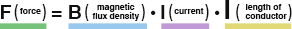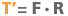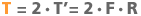﻿ The Basics of Electromagnetic Forces | Products Information | MABUCHI MOTOR CO., LTD.

# The Basics of Electromagnetic Forces

## Electromagnetic Force

The direction of magnetic flux produced by a permanent magnet is always from N-pole to S-pole.
When a conductor is placed in a magnetic field and current flows in the conductor, the magnetic field and the current interact each other to produce force. The force is called "Electromagnetic force".

The fleming's left hand rule determines the direction of the current, the magnetic force and the flux. Stretch the thumb, the index finger and the middle finger of your left hand as shown in Fig. 2.

When the middle finger is the current and the index finger the magnetic flux, the direction of the force is given by the thumb.

## Magnet field produced by current

The magnetic fields produced by the current and the permanent magnets works to produce electromagnetic force.
When the current flows in the conductor toward the reader, the magnetic field in the CCW direction will be produced around the current flow by the right-handed screw rule (Fig.3).

## Interference of a line of magnetic force

The magnetic fields produced by the current and the permanent magnets interfere each other.
The line of magnetic force distributed in the same direction acts to increase its strength, while the flux distributed in the opposite direction acts to reduce its strength.

## Electromagnetic force production

The line of magnetic force has a nature to return to the straight line by its tension like an elastic band.
Thus, the conductor is forced to move from where the magnetic force is stronger to where it is weaker (Fig.5).

## Torque production

Electromagnetic force is obtained from the equation;Fig.6 illustrates the torque obtained when a single-turn conductor is placed in the magnetic filed.
The torque produced by the single conductor is obtained from the equation;• T'(torque)
• F (force)
• R (distance from the center to conductor)

Here, there are two conductors present;